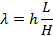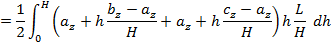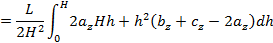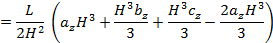## Saturday, October 1, 2011

### Volume of Irregular Triangular Prism

A triangular prism is a 3 dimensional shape with a triangular cross-section.  By “irregular triangular prism” I mean a triangular prism where the ends are not both parallel with a cross-section of the prism. (If you're interested in a "really irregular triangular pyramid", try here.)  The below pictures demonstrate:

In the above picture, a, b, and c are parallel sides, the base B is parallel with the cross-section, but the top end is not.  (If both ends are not parallel with the cross-section, we still name it the same way.)  In a previous post, I indicated that the volume of this rascal is given by V = (a+b+c)B/3, where B is the area of the cross-section.  Today, it’s time to prove it.  We do so using integration.

We first observe, that the orientation of the object does not affect is volume.  We may therefore move (translate and rotate) any given prism (as above) so that the sides a, b, and c are parallel with z-axis and cross-sections are parallel with the xy-plane.
We first consider the shape above with base B coincident with the xy-plane and denote the top end points of sides a, b, and c according to the pattern a = (ax, ay, az).  We will only need the z-coordinates.  The top view of the above shape looks like this:

Notice the dh in the picture above.  It represents the thickness of a slice of our prism.  The zL and zR represent the height of the sides of the slice.  (We could have used the other side of the slice if we wanted – what matters is consistency.)  Notice that our slices have a rectangular top view but a trapezoidal side view - the left and right sides are different elevations (z-coordinates).  Hence, we may take dV = ½(zL+ zR) λ dh.  Note that zL, zR, and λ, are functions of h as follows:Therefore,If the bottom end of the prism is not parallel with the xy-plane, we move it above the xy-plane and determine the volume of the shape using subtraction of volumes.  We write az, bz, cz, as the z-coordinates of the top of lines a, b, and c, and az’, bz’, and cz’ for the bottom end points.  Now, V = Vtop – Vbottom, where Vtop is the volume below the top end and Vbottom is the volume below the bottom end.  This becomes V = (az + bz + cz)B/3 – (az’ + bz’ + cz’)B/3 = ((az – az’) + (bz – bz’) + (cz – cz’))B/3 = (a + b + c)B/3, where in this case a = az – az’, b = bz – bz’, and c = cz – cz’.  This completes the argument.